0%

# 电化学系统中的等效电路

## Randles等效电路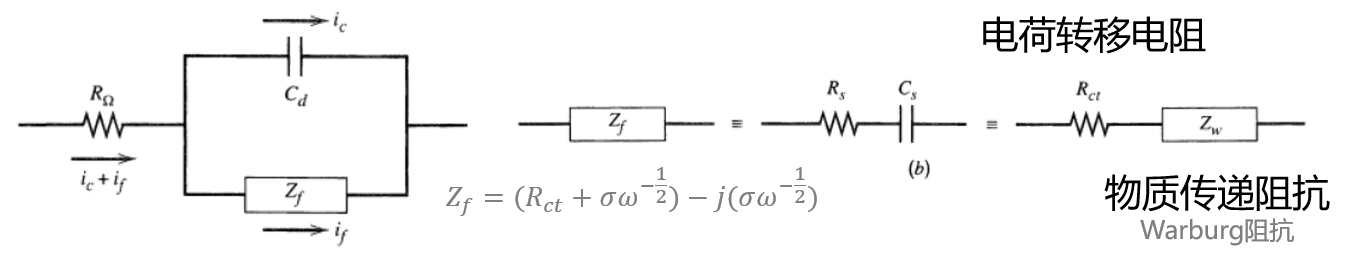Randles等效电路

“等效”不太一样。我们不清楚电路的具体模型是什么，因此我们假设了一个，对不对我们也不知道。有的时候能够描述比较好，而有的时候不行。因此不代表我们可以用足够复杂的电路去描述一个电化学系统。

Randles等效电路远非随意假设。在电化学分析中，我们最关注的是化学过程法拉第过程在电极与电解质之间产生了电子转移，在不同物质之间发生了氧化还原，是我们电化学分析中着重关注的过程。这一过程对应的阻抗即法拉第阻抗$Z_f$，也是我们后来理论推导中的着力点。

## 电解质电阻与双电层电容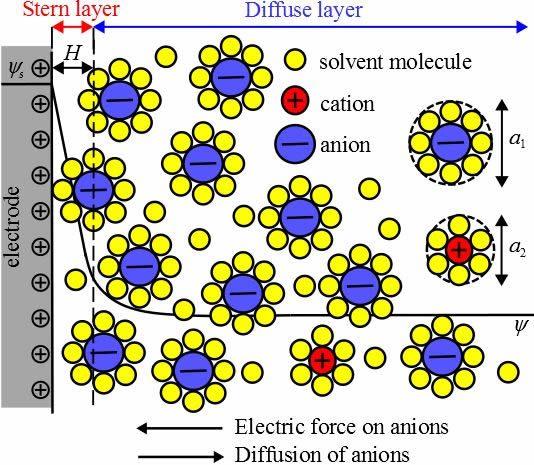Ref: https://www.seas.ucla.edu/~pilon/EDLCs.htm

## 如果是纯粹物理电路

$$\frac{\text{d}E}{\text{d}t} = \left( R_sI\omega \right) \cos(\omega t) + \left( \frac{I}{C_s} \right) \sin(\omega t)$$

## 回到现实电化学系统

$$\frac{\text{d}E}{\text{d}t} = \frac{\partial E}{\partial i} \frac{\text{d} i}{\text{d} t} + \frac{\partial E}{\partial C_O} \frac{\text{d} C_O}{\text{d} t} + \frac{\partial E}{\partial C_R} \frac{\text{d} C_R}{\text{d} t}$$

## 通过组装$R_s$和$C_s$获得法拉第阻抗$Z_f$

$$\frac{\text{d}E}{\text{d}t} = \left( R_{ct} + \sigma\omega^{-1/2} \right) I\omega \cos(\omega t) + \left( \sigma \omega^{1/2} \right) I \sin(\omega t)$$

$$Z_f = R_s+ C_s = R_s - j \frac{1}{\omega C_s} = R_{ct} + \sigma \omega^{-1/2}-j(\sigma \omega^{-1/2})$$

### 电荷传递阻抗$R_{ct}$和$Warburg$阻抗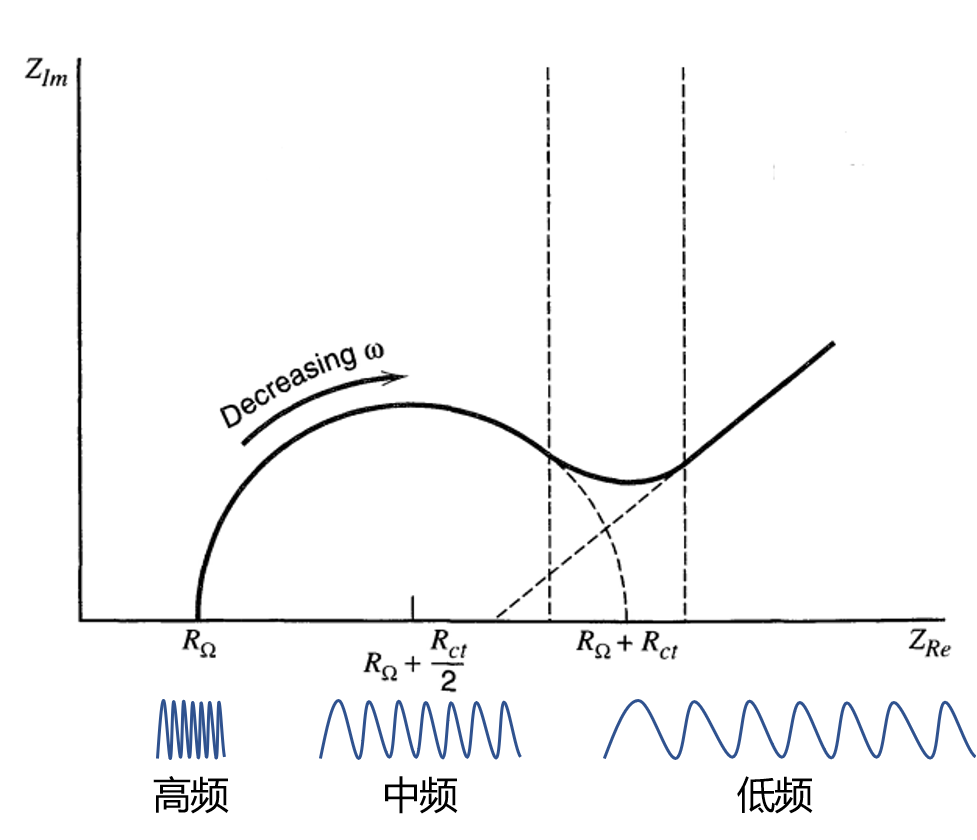Warburg​阻抗（与传质相关）

# 电化学系统总阻抗

（中间省略部分数学处理）且

## 低频极限，$\omega \rightarrow 0$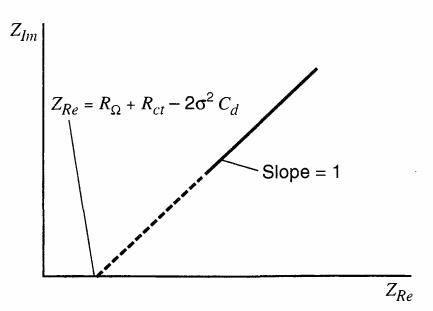## 高频极限，$\omega \rightarrow \infty$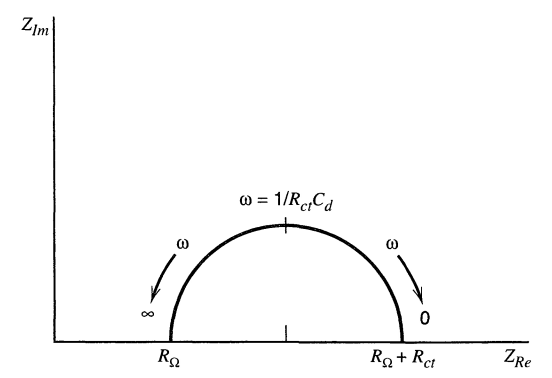## 现实阻抗在两个极限情况之间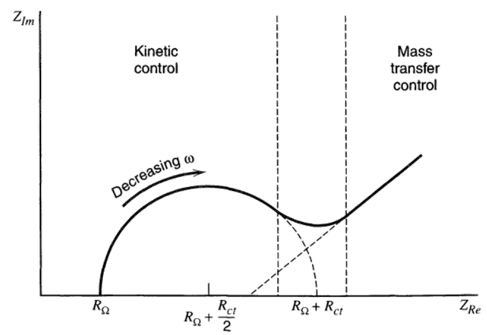# 在能源电化学中的应用实例

• 从以上Nyquist图中不难看出，高频区域的半圆与$R_{Im}$的交点为电解质阻值$R_\Omega$，而其直径为$R_{ct}$[1, 2]。也就是说，半圆直径越小，电子转移阻抗越小，一般也越有利于电化学反应的发生。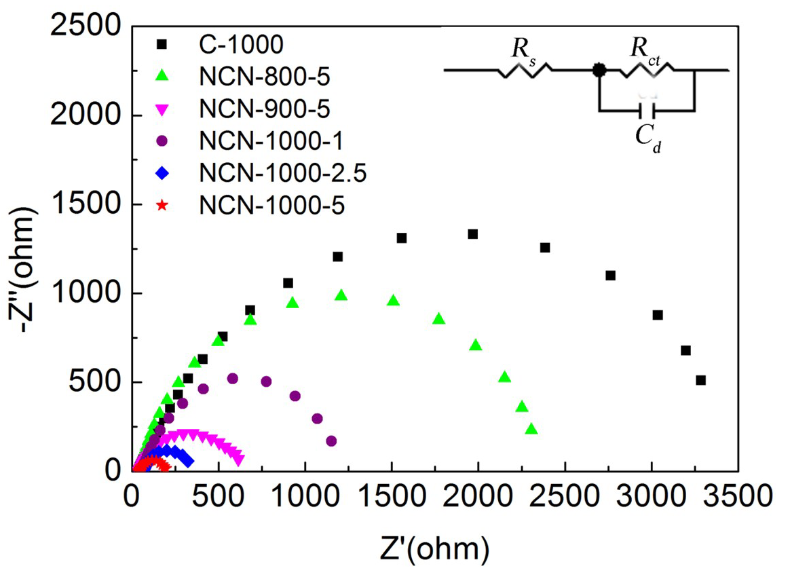Ref: Jiang et al. Defect-Rich and Ultrathin N Doped Carbon Nanosheets as Advanced Trifunctional Metal-Free Electrocatalysts for the ORR, OER and HER. Energy Environ. Sci. 2019, 12 (1), 322–333.

• 低频区域直线的的斜率与离子扩散系数呈线性关系，反映了离子传输阻抗：斜率越大，离子扩散系数越大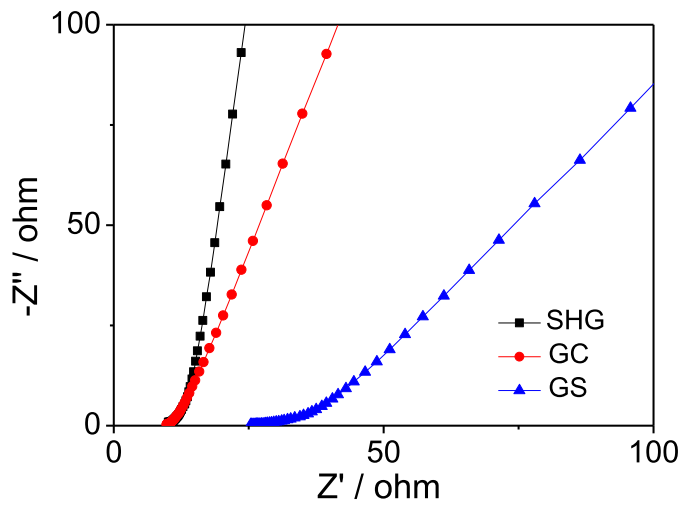Ref: Dai* et al. Multifunctional Carbon-Based Metal-Free Electrocatalysts for Simultaneous Oxygen Reduction, Oxygen Evolution, and Hydrogen Evolution. Adv. Mater. 2017, 29 (9), 1604942.

• 高频和中频区域可能出现两个半圆。其中前一个半圆（高频）对应钝化膜形成阻抗（passivated film impedance），后一个半圆（中频）对应电荷转移阻抗（charge-transfer resistance）。这种半圆或圆弧的数目与等效电路中的电容个数直接相关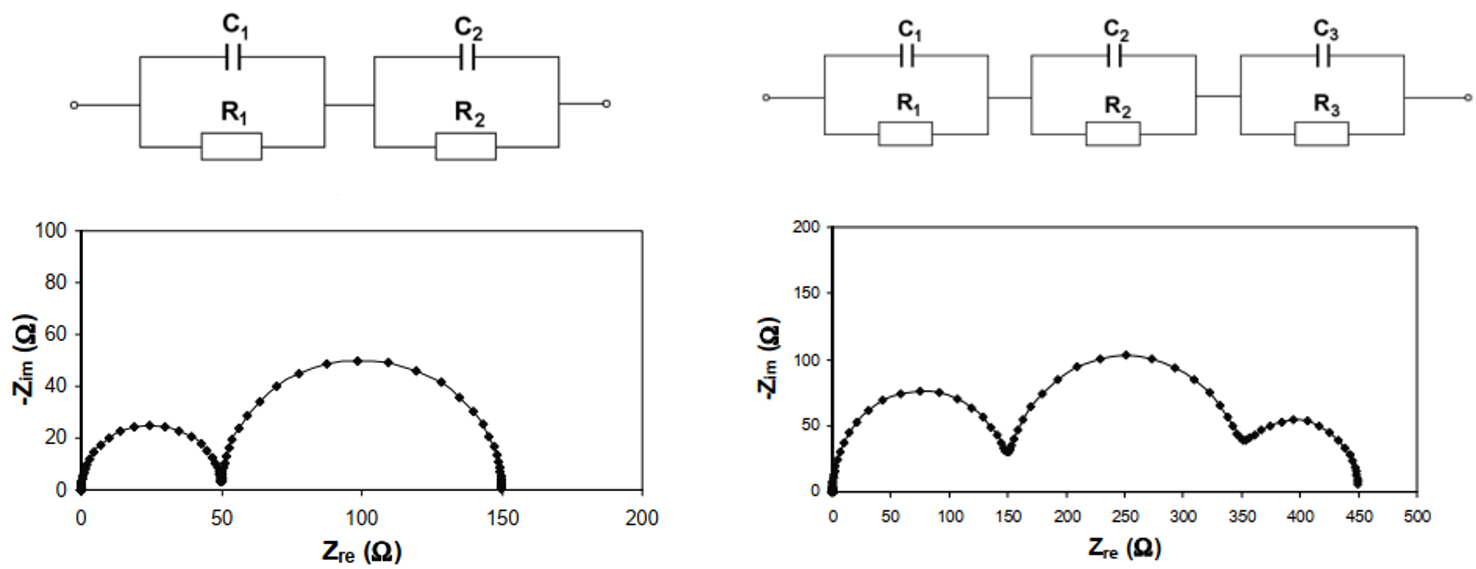Ref: Yuan et al. Electrochemical Impedance Spectroscopy in PEM Fuel Cells; 2010.

# 参考文献

 Bard, A. J., Faulkner, L. R., Leddy, J., & Zoski, C. G. (1980). Electrochemical methods: Fundamentals and applications (Vol. 2). New York: wiley.

 Yang, X.; Rogach, A. L. Electrochemical Techniques in Battery Research: A Tutorial for Nonelectrochemists. Adv. Energy Mater. 2019, 9 (25), 1900747.

 Dai* et al. Multifunctional Carbon-Based Metal-Free Electrocatalysts for Simultaneous Oxygen Reduction, Oxygen Evolution, and Hydrogen Evolution. Adv. Mater. 2017, 29 (9), 1604942.

 (a) Mirzaeian M, Hall P J. Characterizing capacity loss of lithium oxygen batteries by impedance spectroscopy [J]. J Power Sources, 2010, 195: 6817-6824.(b) Song J Y, Lee H H, Wang Y Y, et al. Two- and three-electrode impedance spectroscopy of lithium-ion batteries [J]. J Power Sources, 2002, 111: 255-267. (c) Chang Y C, Sohn H J. Electrochemical impedance analysis for lithium ion intercalation into graphitized carbons [J]. J Electrochem Soc, 2000, 147: 50-58.